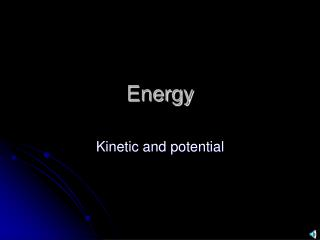Download PresentationEnergyEnergy - PowerPoint PPT Presentation

Download PresentationEnergy
An Image/Link below is provided (as is) to download presentation

Download Policy: Content on the Website is provided to you AS IS for your information and personal use and may not be sold / licensed / shared on other websites without getting consent from its author. While downloading, if for some reason you are not able to download a presentation, the publisher may have deleted the file from their server.

- - - - - - - - - - - - - - - - - - - - - - - - - - - E N D - - - - - - - - - - - - - - - - - - - - - - - - - - -
Presentation Transcript

1. Energy Kinetic and potential

2. ENERGY Standard: S8P2 a.Explain energy transformation in terms of the Law of Conservation of Energy. b. Explain the relationship between potential and kinetic energy.

3. Energy can be classified as potential or kinetic • Potential energy: energy of position The boulder has more gravitational potential energy when measured from point A compared to B. PE = mgh

4. PE = mgh • m = mass in kilograms • g = acceleration due to gravity (9.8 m/s2) • h = height in meters • Potential Energy is measured in kg • m/s2• m = newton meter = Joules

5. Example 1: • If the boulder has a mass of 8kg, and distance B is 10m, what is the potential energy of the boulder relative to the plateau? 8kg 10m

6. Answer: • PE = mgh • = 8kg • 9.8m/s2 • 10m • =784 J 8kg 10m

7. Example 2: • If distance A is 20m, what is the potential energy of the boulder relative to the bottom? 8kg 20m

8. Answer: • PE = mgh • = 8kg • 9.8m/s2 • 20m • = 1568 J 8kg 20m

9. Example 3 • When work is done to stretch something, it is called elastic potential energy. Find the elastic potential energy stored in a drawn bow if it takes an average force of 100N to pull the arrow back a distance of 0.5 meters.

10. Answer: • PE = mgh = Fd Fxd = 100N x 0.5m = 50J

11. Kinetic energy • Energy of motion • KE = ½ mv2

12. KE = 1/2mv2 • m = mass in kilograms • v = velocity in meters/sec • Kinetic energy is measured in • kg • m/s • m/s = • kg m/s2 • m = • newton meter = • Joules

13. Example 4 • The bird has a mass of 2 kg. It is flying at a speed of 5 m/s. Find its kinetic energy. • KE = ½ mv2 • = ½ • 2kg • (5m/s)2 • = ½ • 2kg • 25 m2/s2 • = 25 joules

14. Kinetic and potential energy conversions • Describe the energy conversions in this picture: • At the top: • ¼ of the way down: • ½ way down: • ¾ down • At the bottom: • Is the sum of KE + PE a constant? All PE, no KE 3/4 PE, 1/4 KE 1/2 PE, 1/2 KE 1/4 PE, 3/4 KE No PE, All KE Yes! It’s always 10,000 J in this case.

15. Where are PE and KE maximums in this picture? PE and KE Max PE no KE Max KE No PE Max PE No KE

16. What happens when the cord is cut? Potential energy is converted to kinetic energy!

17. Kinetic and potential energy convert to one another PE max PE max no KE no KE KE max KE max no PE no PE

18. How is mass related to energy? • Albert gave us the answer! E=mc2

19. E=mc2 • E = energy in joules • m = mass in kilograms • c = speed of light • (3 x 108 m/s)

20. A small mass gives a great amount of energy

21. Nuclear energy- clean power for the world?

22. Example: • How much energy can be given off by 5 grams of mass? ( 10 paper clips = 5 g) • 5g = 0.005 kg • E=mc2 • = 0.005kg x (3 x 108 m/s)2 • = 0.005 kg x 9 x 1016 m2/s2 • = 0.045 x 1016 • = 450,000,000,000,000 J

23. WOW!!! • 450,000,000,000,000 J is the amount of energy in 3 million gallons of gasoline!

24. Einstein's theories of relativity have been proved during solar eclipses. Light is bent by gravity

25. See ya! The End